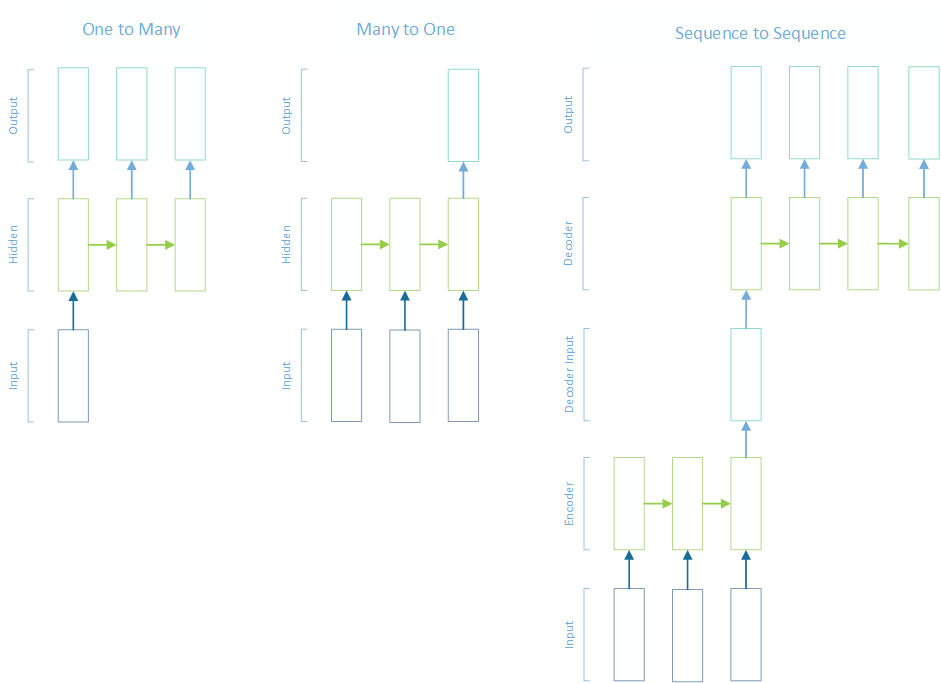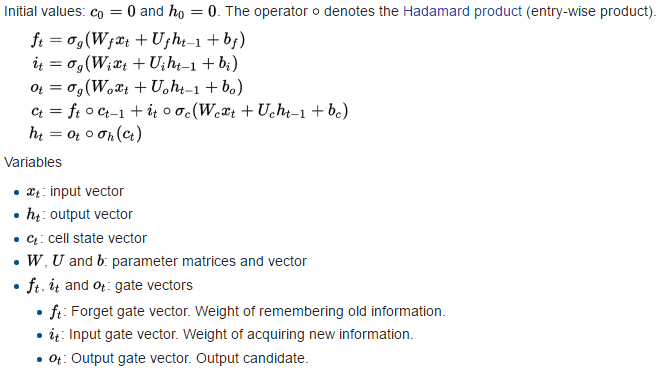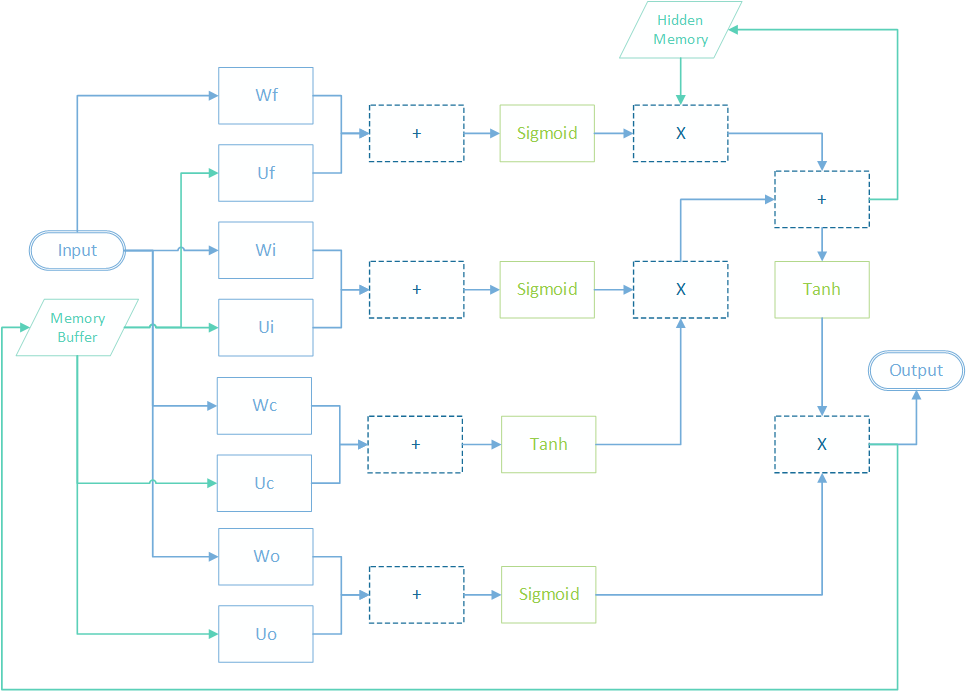Using different recurrent neural network architectures for classifying sequential inputs such as one to many, many to one and sequence to sequence with Long Short Term Memory (LSTM)

Motivation

For machine learning tasks involved with classifying sequences of data there might not be a one to one mapping between input and output classifications. For example, when building neural networks that learn to translate between different languages it is unlikely that there will be the same number of words in every translated sentence compared to each input sentence.

One way the output can be varied in length against the input is with something called a sequence to sequence (STS) recurrent neural network. This is when the network is divided into two separate classifiers, one called an encoder and the other the decoder.

The encoder is tasked with learning to generate a single embedding that effectively summarises the input and the decoder is tasked with learning to generate a sequence of output from that single embedding.

So a sequence to sequence architecture is actually a combination of two other architectures - one to many and many to one, each of which are useful on their own in other learning tasks.Long Short Term Memory

Long Short Term Memory (LSTM) networks are a recurrent neural network that can be used with STS neural networks. They are similar to Gated Recurrent Units (GRU) but have an extra memory state buffer and an extra gate which gives them more parameters and hence a longer training time. While performance with GRU is usually comparable, there are some tasks that each architecture outperforms the other on, so comparing each for a given learning task is usually a good idea.

LSTM networks are implemented with:Since Bright Wire is a graph oriented machine learning framework, the graph for the LSTM network is implemented as:As with a GRU, the memory buffer is updated with the layer's output at each step in the sequence, and then this saved output flows into the next item in the sequence. Unlike a GRU, LSTM networks have an additional memory state that is updated after each pass through the network in the same way.

Many to One

The first part of a STS architecture is the encoder that learns how to encode the most relevant parts of a sequence of input into a single embedding. While used in STS, this architecture is actually useful on its own.

For example, when comparing documents for similarity one approach is to create a document embedding for each document in a set of documents and then use a distance metric such as euclidean distance to compare documents to each other. A many to one recurrent neural network is one way to obtain such document embeddings.

Another example would be classifying sentences as either positive or negative sentiment. The single output label "positive" might apply to an entire sentence (which is composed of a sequence of words).

In Bright Wire, a many to one training set is a data table with a matrix input column and a vector output column. The matrix contains the sequence of input vectors and the output contains the target output vector.

To keep things simple in this tutorial, our input matrix will contain a sequence of five one-hot encoded characters and the output vector will contain a union of those characters. So for example if our sequence is {"A", "B", "D"} the first vector will contain "A", the second "B" and the third "D" and the output vector will be "ABD". In this case the encoder just needs to keep track of all the characters that it has seen and write them into the output vector.

We can create training and test data sets with a SequenceClassification helper class in Bright Wire. Our dictionary size (the number of possible characters) is 10, and each generated sequence is of length 5. Also, no character will repeat in any generated sequence. 1000 sequences are generated and split into training and test sets.

var grammar = new SequenceClassification(dictionarySize: 10, minSize: 5, maxSize: 5, noRepeat: true, isStochastic: false);
var sequences = grammar.GenerateSequences().Take(1000).ToList();
var builder = BrightWireProvider.CreateDataTableBuilder();

foreach (var sequence in sequences) {
var list = new List<FloatVector>();
var charSet = new HashSet<char>();
foreach (var ch in sequence) {
var row = grammar.Encode(charSet.Select(ch2 => (ch2, 1f)));
}
}
var data = builder.Build().Split(0);

Next, the LSTM network is trained for 10 iterations using rms prop gradient descent and a hidden memory size of 128. The binary classification error metric rounds outputs to either 0 or 1.

using (var lap = BrightWireProvider.CreateLinearAlgebra(false)) {
var graph = new GraphFactory(lap);
var errorMetric = graph.ErrorMetric.BinaryClassification;

// create the property set
var propertySet = graph.CurrentPropertySet
.Use(graph.WeightInitialisation.Xavier)
;

// create the engine
var trainingData = graph.CreateDataSource(data.Training);
var testData = trainingData.CloneWith(data.Test);
var engine = graph.CreateTrainingEngine(trainingData, 0.03f, 8);

// build the network
const int HIDDEN_LAYER_SIZE = 128;
var memory = new float[HIDDEN_LAYER_SIZE];
var network = graph.Connect(engine)
;

engine.Train(10, testData, errorMetric);

var networkGraph = engine.Graph;
var executionEngine = graph.CreateEngine(networkGraph);

var output = executionEngine.Execute(testData);
Console.WriteLine(output.Where(o => o.Target != null).Average(o => o.CalculateError(errorMetric)));
}

Since this is such a simple learning task, the network very quickly reaches 100% accuracy.

One to Many

One to many neural networks generate a sequence of output from a single input vector. An example of this architecture might be learning to generate a sequence of commands after observing the current state of a system.

A one to many training data set is created with a data table that contains a vector input column and matrix output column. Along the same lines as the many to one example above, the following code creates a vector that summarises a sequence and the sequence itself encoded as one hot vectors.

var grammar = new SequenceClassification(dictionarySize: 10, minSize: 5, maxSize: 5, noRepeat: true, isStochastic: false);
var sequences = grammar.GenerateSequences().Take(1000).ToList();
var builder = BrightWireProvider.CreateDataTableBuilder();

foreach (var sequence in sequences) {
var sequenceData = sequence
.GroupBy(ch => ch)
.Select(g => (g.Key, g.Count()))
.ToDictionary(d => d.Item1, d => (float)d.Item2)
;
var summary = grammar.Encode(sequenceData.Select(kv => (kv.Key, kv.Value)));
var list = new List<FloatVector>();
foreach (var item in sequenceData.OrderBy(kv => kv.Key)) {
var row = grammar.Encode(item.Key, item.Value);
}
}
var data = builder.Build().Split(0);

In this case, it's a harder problem than the many to one as the neural network needs to learn the correct order of each output character (an "A" before a "D" etc). It takes around 50 epochs to converge and reaches 99.95% accuracy.

In this case the training rate is reduced at the 30th epoch to try to improve the accuracy in the later stages of training.

using (var lap = BrightWireProvider.CreateLinearAlgebra(false)) {
var graph = new GraphFactory(lap);
var errorMetric = graph.ErrorMetric.BinaryClassification;

// create the property set
var propertySet = graph.CurrentPropertySet
.Use(graph.WeightInitialisation.Xavier)
;

// create the engine
const float TRAINING_RATE = 0.1f;
var trainingData = graph.CreateDataSource(data.Training);
var testData = trainingData.CloneWith(data.Test);
var engine = graph.CreateTrainingEngine(trainingData, TRAINING_RATE, 8);
engine.LearningContext.ScheduleLearningRate(30, TRAINING_RATE / 3);

// build the network
const int HIDDEN_LAYER_SIZE = 128;
var memory = new float[HIDDEN_LAYER_SIZE];
var network = graph.Connect(engine)
;

engine.Train(40, testData, errorMetric);

var networkGraph = engine.Graph;
var executionEngine = graph.CreateEngine(networkGraph);

var output = executionEngine.Execute(testData);
Console.WriteLine(output.Average(o => o.CalculateError(errorMetric)));
}

Sequence to Sequence

The simplest type of STS network is a recurrent auto encoder. In this scenario, the encoder is learning to encode an input sequence into an embedding and the decoder is learning to decode that embedding into the same input sequence.

In a recurrent auto encoder the input and output sequence lengths are necessarily the same, but we are using the encoder's ability to find the relevant discriminative features of the input as it creates the single embedding from the input sequence.

Once the network has converged, we can throw the decoder away and use the encoder to create sequence embeddings. This is how we might build a single embedding from a sequence of words (the document) for the purposes of document comparison.

But purely to demonstrate that the input and output sequences can in fact be different we will be discarding the last item in each sequence in this tutorial when training the decoder. So the output sequence for this tutorial will always be shorter than the input sequence.

A STS data set in Bright Wire is a data table with two Matrix columns. The matrices each contain rows that form the input and output sequences. Obviously for sequence to sequence, those vectors do not need to contain the same number of rows.

As STS networks have been shown to perform better with reversed output, the input sequences are reversed for the decoder output.

const int SEQUENCE_LENGTH = 5;
var grammar = new SequenceClassification(8, SEQUENCE_LENGTH, SEQUENCE_LENGTH, true, false);
var sequences = grammar.GenerateSequences().Take(2000).ToList();
var builder = BrightWireProvider.CreateDataTableBuilder();

foreach (var sequence in sequences) {
var encodedSequence = grammar.Encode(sequence);
var reversedSequence = new FloatMatrix {
Row = encodedSequence.Row.Reverse().Take(SEQUENCE_LENGTH - 1).ToArray()
};
}
var data = builder.Build().Split(0);

In Bright Wire, the decoder and encoder are defined in two separate graphs that are stitched together to create the sequence to sequence architecture. The input is executed by the first graph, then the single output vector is passed to the second graph. The second graph generates its output and then backpropagates its error back to the first graph.

To give the decoder more data to work with, it's possible to append the encoder's internal memory buffer with the encoder's output. This is done by writing the encoder's memory state to a named memory slot on every iteration and then joining that memory with the encoder's output data in the decoder.

The network configuration is much the same as the one to many and many to one networks above.

using (var lap = BrightWireProvider.CreateLinearAlgebra()) {
var graph = new GraphFactory(lap);
var errorMetric = graph.ErrorMetric.BinaryClassification;

// create the property set
var propertySet = graph.CurrentPropertySet
.Use(graph.WeightInitialisation.Xavier)
;

const int BATCH_SIZE = 16;
int HIDDEN_LAYER_SIZE = 64;
const float TRAINING_RATE = 0.1f;

// create the encoder
var encoderLearningContext = graph.CreateLearningContext(TRAINING_RATE, BATCH_SIZE, TrainingErrorCalculation.Fast, true);
var encoderMemory = new float[HIDDEN_LAYER_SIZE];
var trainingData = graph.CreateDataSource(data.Training, encoderLearningContext, wb => wb
.WriteNodeMemoryToSlot("shared-memory", wb.Find("encoder") as IHaveMemoryNode)
);
var testData = trainingData.CloneWith(data.Test);

// create the engine
var engine = graph.CreateTrainingEngine(trainingData, TRAINING_RATE, BATCH_SIZE);
engine.LearningContext.ScheduleLearningRate(30, TRAINING_RATE / 3);
engine.LearningContext.ScheduleLearningRate(40, TRAINING_RATE / 9);

// create the decoder
var decoderMemory = new float[HIDDEN_LAYER_SIZE];
var wb2 = graph.Connect(engine);
wb2
.JoinInputWithMemory("shared-memory")
.IncrementSizeBy(HIDDEN_LAYER_SIZE)